Download Presentation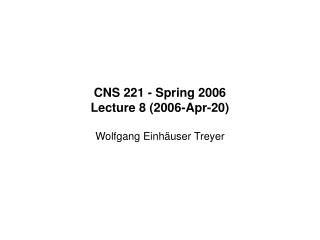CNS 221 - Spring 2006 Lecture 8 (2006-Apr-20) Wolfgang Einhäuser Treyer

# CNS 221 - Spring 2006 Lecture 8 (2006-Apr-20) Wolfgang Einhäuser Treyer - PowerPoint PPT Presentation

CNS 221 - Spring 2006 Lecture 8 (2006-Apr-20) Wolfgang Einhäuser Treyer Even for constant stimulus, neurons fire irregularly Sources of noise At individual neuron (“intrinsic”) thermal noise: <V 2 > ~ R k B T finite number of channels in membrane - channels are either open or closedI am the owner, or an agent authorized to act on behalf of the owner, of the copyrighted work described.
Download Presentation## CNS 221 - Spring 2006 Lecture 8 (2006-Apr-20) Wolfgang Einhäuser Treyer

An Image/Link below is provided (as is) to download presentation

Download Policy: Content on the Website is provided to you AS IS for your information and personal use and may not be sold / licensed / shared on other websites without getting consent from its author.While downloading, if for some reason you are not able to download a presentation, the publisher may have deleted the file from their server.

- - - - - - - - - - - - - - - - - - - - - - - - - - E N D - - - - - - - - - - - - - - - - - - - - - - - - - -
Presentation Transcript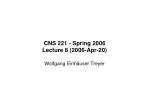CNS 221 - Spring 2006

Lecture 8 (2006-Apr-20)

Wolfgang Einhäuser Treyer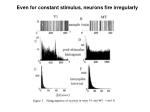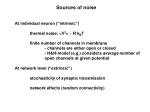Sources of noise

At individual neuron (“intrinsic”)

thermal noise: <V2> ~ R kBT

finite number of channels in membrane

- channels are either open or closed

- H&H-model (e.g.) considers average number of open channels at given potential

At network level (“extrinsic”)

stochasticity of synaptic transmission

network effects (random connectivity)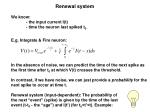Renewal system

We know:

- the input current I(t)

- time the neuron last spiked t0

E.g. Integrate & Fire neuron:

In the absence of noise, we can predict the time of the next spike as the first time after t0 at which V(t) crosses the threshold.

In contrast, if we have noise, we can just provide a probability for the

next spike to occur at time t.

Renewal system (input-dependent): The probability of

the next “event” (spike) is given by the time of the last

event (t-t0 - the “age”) and I(t’) (for t0<t’<t). Example: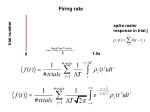Firing rate

spike raster

response in trial j

trial number

t

1.5s

0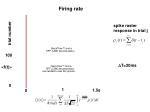Firing rate

spike raster

response in trial j

trial number

100

DT=20ms

<f(t)>

0

t

1.5s

0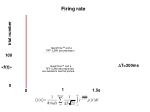Firing rate

trial number

100

DT=200ms

<f(t)>

0

t

1.5s

0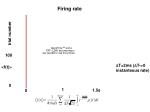Firing rate

trial number

100

DT=2ms (DT->0

instanteous rate)

<f(t)>

0

t

1.5s

0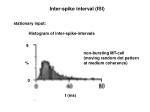Inter-spike interval (ISI)

stationary input:

Histogram of inter-spike-intervals

9

non-bursting MT-cell

(moving random dot pattern

at medium coherence)

%

0

t (ms)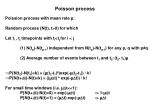Poisson process

• Poission process with mean rate µ:
• Random process {N(t), t>0} for which
• Let ti , tj timepoints with ti<tj for i < j
• (1) N(tp)-N(tp-1) independent from N(tq)-N(tq-1) for any p, q with p≠q
• (2) Average number of events between t1 and t2:(t2- t1)µ
• P{N(t2)-N(t1)=k} = (µ(t2-t1)k)exp(-µ(t2-t1)) / k!
• P{N(t+Dt)-N(t)=k} = ((µDt) k exp(-µDt)) / k!

For small time windows (i.e. µDt<<1):

P{N(t+Dt)-N(t)=0} = exp(-µDt) -> 1-µDt

P{N(t+Dt)-N(t)=1} = (µDt) exp(-µDt) -> µDtPoisson process

For small time windows (i.e. µDt<<1):

P{N(t+Dt)-N(t)=0} = 1-µDt

P{N(t+Dt)-N(t)=1} = µDt

So:

- choose Dt small, that maximally 1 spike happens in Dt,

- bin your time into intervals of Dt

- for each bin draw a random variable 0<r<1 from a uniform distr.

- spike if r ≤ µDtPoisson process

P{N(t+Dt)-N(t)=k} = (µDt)exp(-µDt) / k!

If spike has ocurred at t=0 the probability that no spike has ocuered by time t is given:

P{N(t)-N(0)=0} = exp(-µt)

Hence the probability that at least one spike has ocurred is

1- exp(-µt)

And the probability density

p(t) = µexp(-µt)

So in terms of ISIs:Given we had a spike at t0 the probability to have another spike at t is p(t|t0) = µexp(-µ(t-t0))Poisson process

Mean firing rate:

Mean interval between spikes:

So the mean firining rate is indeed µ, as intendedInter-spike interval (ISI)

9

%

p

0

refractory

period !

t (ms)

3/µ

0

1/µ

tNoise spectrum

Powerspectrum:

Relation to autocorrelation:

Fourier transform of Cii(s) is P(w)Normalized Autocorrelation

• Mean firing rate: µ
• probability to find spike in [t,t+Dt]: µ Dt
• for large inter-spike distances, expectation for spike

independent of spike long time ago, hence define

• transform to Fourier space
• divergence for w->0 in unnormalized case!Autocorrelation

So far:

p(t) (see interspike interval)

0

t

t

Given we had a spike at t=0, what is the probability for the nextspike at t?

s

Now:

t0

t1

t

What is the probability to find two spikes at distance s

(independent of the spikes between them)?Autocorrelation

Assume we had a spike at time t (which we expect with rate µ),

the probability for a spike at t+s is given by Cii(s) Q(s), hence we can

express the probability to find any two spikes at distance s as C+(s) by

µC+(s) = Cii(s) Q(s)

where µ is the (prior) probability for having a spike at t.

Hence

Or recursively:

2nd spike at s

3rd spike at s

1st spike at sAutocorrelation

with symmetry and trivial autocorrelation at s=0 we have:

Now Fourier transform

and obtain (convolution -> multiplication in Fourier space)Example: Poisson process

By definition, the probability

Hence:

As one would expect, the autocorrelation is flat, with delta peak at DCPoisson Process with refractory period

Add absoulte refractory period to Poisson procees

Using

one obtainsPoisson Process with refractory period

for large w as without refractory period,

for small w however noise is decreased

=>refractoriness makes spiking more regular

for finite DT the mean firing rate is bound, even for r->infCoefficient of variation (CV)

Quantification of variation:

standard deviation of ISI divided by mean

Regular spiking: CV=0

Poisson process (without refractory period): CV=1

with refractory period: CV<1.Random walk model

Perfect integrator, receiving synaptic input from a random process Ne(t):

V(t) = aeNe(t)

nth events are needed to cross threshold:

nth= [Vthresh/ae]+1

If nth=1, the spiking probability (of our postsynaptic cell) is given by the probability of one presynaptic event to occur, 1-exp(-µt).

The average inter-spike interval is given by nth/µ, where µ is again

the presynaptic rate.

And the variance (as the presynaptic spikes are independent) by nth/µ2.

So the CV scales with 1/sqrt(nth), i.e. (given our Vthresh ) we spike more regularly for many small inputs (small ae => large nth), than for few large ones (large ae, => small nth)Random walk model

Now add inhibitory input

V(t) = aeNe(t) - aiNi(t)

with µ=aeµe - aiµi we have

<V(t)> = µt

and with s2 = a2eµe + a2iµi we have

var(V(t)) = s2 t

probability to cross threshold in finite time:

1 if excitation > inhibition (µ>0)

(aeµe/aiµ)vthrehs otherwise (µ<0)Random walk model

• V(t) = aeNe(t) + aiNi(t); excitation>inhibition (µ>0)
• postsynaptic ISI:
• mean: <Tth>=Vth/µ
• variance: var(Tth)= Vth(aeµe + aiµi)/µ3
• Hence
• CV = sqrt((aeµe + aiµi)/ (Vth(aeµe - aiµi)))
• adding inhibition increases variability (small drift, high jitter)
• “balanced” excitation and inhibition

Adding a leak stabilizes the membrane potential <V(t)>~Rµ